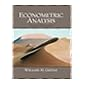Dr. S. R. Lasker Library Online Catalogue

Normal view

# Econometric analysis / William H. Greene.

Material type:TextLanguage: English Series: Pearson series in economicsPublication details: c2012. Edition: 7th ed., international edDescription: 1238 p. : ill. ; 24 cmISBN: 9780273753568; 0273753568Subject(s): EconometricsDDC classification: 330.015195 LOC classification: HB139 | .S688 1995Online resources: Click here to access online
Contents:
TOC Table of Contents Part I: The Linear Regression Model Chapter 1: Econometrics Chapter 2: The Linear Regression Model Chapter 3: Least Squares Chapter 4: The Least Squares Estimator Chapter 5: Hypothesis Tests and Model Selection Chapter 6: Functional Form and Structural Change Chapter 7: Nonlinear, Semiparametric, and Nonparametric Regression Models Chapter 8: Endogeneity and Instrumental Variable Estimation Part II: Generalized Regression Model and Equation Systems Chapter 9: The Generalized Regression Model and Heteroscedasticity Chapter 10: Systems of Equations Chapter 11: Models for Panel Data Part III: Estimation Methodology Chapter 12: Estimation Frameworks in Econometrics Chapter 13: Minimum Distance Estimation and the Generalized Method of Moments Chapter 14: Maximum Likelihood Estimation Chapter 15: Simulation-Based Estimation and Inference Chapter 16: Bayesian Estimation and Inference Part IV: Cross Sections, Panel Data, and Microeconometrics Chapter 17: Discrete Choice Chapter 18: Discrete Choices and Event Counts Chapter 19: Limited Dependent Variables--Truncation, Censoring, and Sample Selection Part V: Time Series and Macroeconometrics Chapter 20: Serial Correlation Chapter 21: Models with Lagged Variables Chapter 22: Time-Series Models Chapter 23: Nonstationary Data Part VI: Appendices Appendix A: Matrix Algebra Appendix B: Probability and Distribution Theory Appendix C: Estimation and Inference Appendix D: Large-Sample Distribution Theory Appendix E: Computation and Optimization Appendix F: Data Sets Used in Applications Appendix G: Statistical Tables
Tags from this library: No tags from this library for this title.
Star ratingsAverage rating: 0.0 (0 votes)
Holdings
Item type Current library Collection Call number Copy number Status Date due Barcode Item holdsText
Reserve Section
Non-fiction 330.015195 GRE 2012 (Browse shelf(Opens below)) C-1 Not For Loan 26928Text
Circulation Section
Non-fiction 330.015195 GRE 2012 (Browse shelf(Opens below)) C-2 Available 26929Text
Circulation Section
Non-fiction 330.015195 GRE 2012 (Browse shelf(Opens below)) C-3 Available 26930
Total holds: 0
##### Browsing EWU Library shelves, Shelving location: Circulation Section Close shelf browser (Hides shelf browser)330.015195 GRE 2003 Econometric analysis / 330.015195 GRE 2003 Econometric analysis / 330.015195 GRE 2003 Econometric analysis / 330.015195 GRE 2012 Econometric analysis / 330.015195 GRE 2012 Econometric analysis / 330.015195 GUB 1988 Basic econometrics / 330.015195 GUB 1988 Basic econometrics /

Includes bibliographic references and index.

TOC Table of Contents Part I: The Linear Regression Model Chapter 1: Econometrics Chapter 2: The Linear Regression Model Chapter 3: Least Squares Chapter 4: The Least Squares Estimator Chapter 5: Hypothesis Tests and Model Selection Chapter 6: Functional Form and Structural Change Chapter 7: Nonlinear, Semiparametric, and Nonparametric Regression Models Chapter 8: Endogeneity and Instrumental Variable Estimation Part II: Generalized Regression Model and Equation Systems Chapter 9: The Generalized Regression Model and Heteroscedasticity Chapter 10: Systems of Equations Chapter 11: Models for Panel Data Part III: Estimation Methodology Chapter 12: Estimation Frameworks in Econometrics Chapter 13: Minimum Distance Estimation and the Generalized Method of Moments Chapter 14: Maximum Likelihood Estimation Chapter 15: Simulation-Based Estimation and Inference Chapter 16: Bayesian Estimation and Inference Part IV: Cross Sections, Panel Data, and Microeconometrics Chapter 17: Discrete Choice Chapter 18: Discrete Choices and Event Counts Chapter 19: Limited Dependent Variables--Truncation, Censoring, and Sample Selection Part V: Time Series and Macroeconometrics Chapter 20: Serial Correlation Chapter 21: Models with Lagged Variables Chapter 22: Time-Series Models Chapter 23: Nonstationary Data Part VI: Appendices Appendix A: Matrix Algebra Appendix B: Probability and Distribution Theory Appendix C: Estimation and Inference Appendix D: Large-Sample Distribution Theory Appendix E: Computation and Optimization Appendix F: Data Sets Used in Applications Appendix G: Statistical Tables

Economics

Saifun Momota

There are no comments on this title.